# Class 10 NCERT Solutions- Chapter 6 Triangles – Exercise 6.5 | Set 2

• Last Updated : 05 Apr, 2021

### Question 11. An aeroplane leaves an airport and flies due north at a speed of 1,000 km per hour. At the same time, another aeroplane leaves the same airport and flies due west at a speed of 1,200 km per hour. How far apart will be the two planes afterhours?

Solution:

Distance covered by place left towards north = 1000 * 1.5

Distance covered by place left towards west = 1200 * 1.5 = 1800km

In right ∆ABC by Pythagoras theorem

(AC)2 = (AB)2 + (BC)2

(AC)2 = (1500)2 + (1800)2

= 250000 + 3240000

= 5490000

= √(3 * 3 * 61 * 10 * 10 * 10 * 10)

= 3 * 10 * 10√61

AC = 300√61

Distance between the two poles 300√61km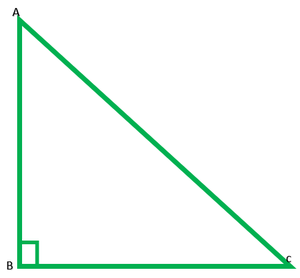### Question 12. Two poles of heights 6 m and 11 m stand on a plane ground. If the distance between the feet of the poles is 12 m, find the distance between their tops.

Solution:

AB is pole of height = 11m

DC is another pole of height = 6m

BC = 12m

In fig.  DE = BC = 12m

AE = AB – EB

= 11 – 6

= 5m

In right ∆AED, by Pythagoras theorem

Hence, the distance between the tops of the two poles is 13m.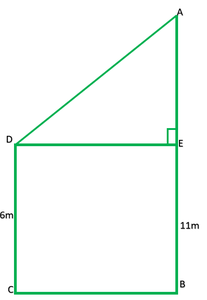### Question 13. D and E are points on the sides CA and CB respectively of a triangle ABC right angled at C. Prove that AE2 + BD2 = AB2 + DE2.

Solution:

To prove: (AE)2 + (BD)2 = (AB)2 + (DE)2

Construction: Join AE, BD and DE

Proof:

In ∆ACE, by Pythagoras theorem

(AE)2 = (AC)2 + (EC)2          -(1)

In ∆DCB, by Pythagoras theorem

(BD)2 = (DC)2 + (BC)2          -(2)

In ∆ACB, by Pythagoras theorem

(AB)2 = (AC)2 + (CB)2          -(3)

In ∆DCE, by Pythagoras theorem

(ED)2 = (DC)2 + (CE)2

(AE)2 + (BD)2 = (AC)2 + (EC)2 + (DC)2 + (DC)2

= (AC2 + BC2) + (EC2 + DC2)

= AB2 + DE2          -(from 3 and 4)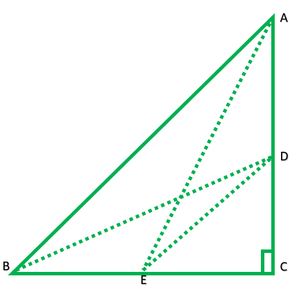### Question 14. The perpendicular from A on side BC of a Δ ABC intersects BC at D such that DB = 3CD (see Fig.). Prove that 2AB2 = 2AC2 + BC2.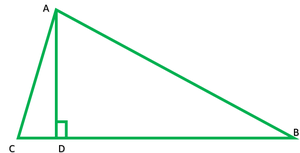Solution:

Given: DB = 3CD

To prove: 2AB2 = 2AC2 + BC2

Proof: DB = 3CD

BC = CD + DB

BC = CD + 3CD

BC = 4CD

BC/4 = CD           -(1)

DB = 3(BC/4)           -(2)

In right ∆ADB, by Pythagoras theorem

AB2 = AD2 + DB2           -(3)

In right ∆ADC, by Pythagoras theorem

AC2 = AD2 + CD2           -(4)

Subtract eq(3) from (4)

= DB2 – CD2

= (3/4BC)2 – (BC/4)2

= 9/6BC2 – BC/16

= 8BC2/16

= AB2 – AC2 = BC2/2

2AB2 – 2AC2 = BC2

2AB2 = 2AC2 + BC2

### Question 15.  In an equilateral triangle ABC, D is a point on side BC such that BD = 1/3BC. Prove that 9AD2 = 7AB2.

Solution:

Construction: Join AD and draw AE perpendicular BC

Let each side, AB = AC = BC = a

BD = 1/3BC = 1/3a

BC = 1/2BC = 1/2a

DE = BE – BD

= 1/2a – 1/3a

= 3a – 2a/6

= DE = a/6

In right ∆AED, by Pythagoras theorem

= 3a2/4+ a2/36

= 27a2/36 + a2/36### Question 16. In an equilateral triangle, prove that three times the square of one side is equal to four times the square of one of its altitudes.

Solution:

Proof: Let each side of one equilateral ∆ = a

BD = 1/2a          -[perpendicular bisects the side in on  an equilateral ∆]

In right ∆ADB, by Pythagoras theorem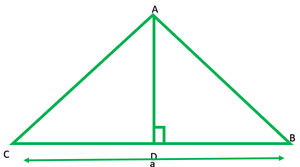### (C) 90°            (D) 45°

Solution:

(AC)2 = (12)2 = 144

(AB)2 = (6√3)2 = 6 * 6√3 = 36 * 3 = 108

(BC)2 = (6)2 = 36

(AB)2 + (BC)2 = 108 + 36 = 144

∴ It is right ∆ thus ∠B = 90°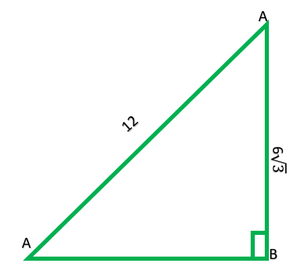My Personal Notes arrow_drop_up